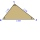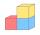# Square root + triangle - math problems

#### Number of problems found: 138

• A rectangle 2A rectangle has a diagonal length of 74cm. Its side lengths are in ratio 5:3. Find its side lengths.
• Secret treasureScouts have a tent in the shape of a regular quadrilateral pyramid with a side of the base 4 m and a height of 3 m. Find the container's radius r (and height h) so that they can hide the largest possible treasure.
• The sides 2The sides of a trapezoid are in the ratio 2:5:8:5. The trapezoid's area is 245. Find the height and the perimeter of the trapezoid.
• Find parametersFind parameters of the circle in the plane - coordinates of center and radius: ?
• CincinnatiA map is placed on a coordinate grid. Cincinnati located at (5,4) and San Diego is located at (-10, -3). How far apart is Cincinnati from San Diego on the map? Round to the nearest tenth.
• Triangular pyramidWhat is the volume of a regular triangular pyramid with a side 3 cm long?
• One trapeziumOne trapezium has AB=24M, BC=36M, CD=80M, DA=80M long sides. Find the area.
• Cube diagonalsCalculate the length of the side and the diagonals of the cube with a volume of 27 cm3.Adam placed the ladder of the house, the upper end reaching to the window at the height of 3.6m, and the lower end standing on level ground and was distant from a wall of 1.5m. What is the length of the ladder?
• Quarter circleWhat is the radius of a circle inscribed in the quarter circle with a radius of 100 cm?
• Pyramid cutWe cut the regular square pyramid with a parallel plane to the two parts (see figure). The volume of the smaller pyramid is 20% of the volume of the original one. The bottom of the base of the smaller pyramid has a content of 10 cm2. Find the area of the
• Two peopleTwo straight lines cross at right angles. Two people start simultaneously at the point of intersection. John walking at the rate of 4 kph in one road, Jenelyn walking at the rate of 8 kph on the other road. How long will it take for them to be 20√5 km apa
• Is right-angledCan a triangle with the sides of sqrt 3, sqrt 5 and sqrt 8 (√3, √5 and √8) be a right triangle?
• ABCD squareIn the ABCD square, the X point lies on the diagonal AC. The length of the XC is three times the length of the AX segment. Point S is the center of the AB side. The length of the AB side is 1 cm. What is the length of the XS segment?
• CalculateCalculate the length of the wall diagonal of the cube with an edge 5 cm long.The ladder is 10 m long The ladder is 8 m high How many meters is the distant heel from the wall?
• Body diagonalCuboid with base 7cm x 3,9cm and body diagonal 9cm long. Find the height of the cuboid and the length of the diagonal of the base,
• Triangle KLMIn the rectangular triangle KLM, where is hypotenuse m (sketch it!). Find the length of the leg k and the height of triangle h if the hypotenuse's segments are known MK = 5cm and ml = 15 cm.
• Cube and sphereCube with the surface area 150 cm2 is described sphere. What is sphere surface?Ladder 10 meters long is staying against the wall so that its bottom edge is 6 meters away from the wall. What height reaches ladder?